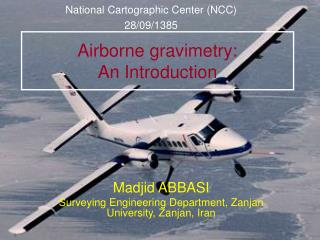Download PresentationAirborne gravimetry: An Introduction

# Airborne gravimetry: An Introduction

Download Presentation## Airborne gravimetry: An Introduction

- - - - - - - - - - - - - - - - - - - - - - - - - - - E N D - - - - - - - - - - - - - - - - - - - - - - - - - - -
##### Presentation Transcript

1. National Cartographic Center (NCC) 28/09/1385 Airborne gravimetry:An Introduction Madjid ABBASI Surveying Engineering Department, Zanjan University, Zanjan, Iran

2. Principle of airborne gravimetry

3. Why airborne gravimetry? • Inaccessible regions • Medium wavelengths • Quasi-regular measurements • Cost and time

4. Instrumentation I. GPS multi-receiver configuration II. Gravimetric sensor • Strapdown Inertial Navigation System (INS) • Gravimeter+stabilized platform III. Radar altimeter IV. INS

5. Observatoire Midi-Pyrénées A new approach for data reduction in airborne LaCoste & Romberg gravimetry M. ABBASI, J.P.Barriot (1), J. Verdun and H. Duquenne (2) Bureau Gravimétrique International, Toulouse, France Ecole Nationale des Sciences Géographiques, Paris, France

6. L&R Air/Sea gravimetric system Gravity sensor unit Two axis stabilized platform +

7. Gravity sensor unit Spring Proof mass B Beam Centre of mass of the sensor

8. Fundamental equation of mobile gravimetry : Mathematical modelling Northwards Gravity sensor unit Frame of the gravimeter Inertial system Geocenter

9. Differential equation of the gravimeter This equation relies the gravimetric measurements (S, BandCC) and the accelerations experienced by the gravimeter. The only unknown in this equation is

10. is a low frequency signal Inconvenients of direct computation • The differentiation is not a bounded operator • The frequency content of each term is different Power spectrum of spring tension Power spectrum of cross-coupling effect 200 s 20 s Line number Line number Frequency (Hz) Frequency (Hz)

11. 10 s 200 s Low pass filtering • we know the filtering cut-off frequency just approximately • the filter is applied separately to each acquisition line • …

12. Our new approach: integral equation of the gravimeter We transform the differential equation into an integral equation:

13. Fredholm integral equation of the first kind W Intrinsically ill-posed problem Regularization

14. x ……. the gravity disturbance vector for all the profiles Qx...... the a priori covariance matrix of the unknowns for all the profiles regularization matrix Qy ….. the covariance matrix related to the observations The least squares solution For each profile: Regularization parameter

15. Helmert method The ‘L’ curve method Choice of the regularization parameter Both methods give almost the same results.

16. The a priori covariance matrix of the gravity disturbances Using the existing geopotential models: Correlogram Positive definite function Isotropic covariance function

17. Numerical results of the airborne gravimetric project over the Alps • Number of data ……………………. 103190 • Mean altitude ……………………… 5200 m • Number of North-South lines …..…. 22 • Number of East-West lines ……….. 16 • Mean distance between the lines … 9.6 km

18. Gravity disturbance obtained from the direct filtering Histogramme des écarts aux nœuds avant l’ajustement std = 25 mGal Histogram of the crossover discrepancies std = 16 mGal Exponential filter (resolution 8.4km)

19. Gravity disturbance obtained from the new method Correlations between the gravity disturbances are taken into account via the regularization matrix. Histogramme des écarts aux nœuds avant l’ajustement std = 3 mGal Histogram of the crossover discrepancies std = 2.7 mGal Integral equation method

20. Standard deviation of the estimated gravity disturbances The a posteriori covariance matrix of the estimated gravity disturbances: A criterion for the «precision» of the results The standard deviations decrease around the crossover points and increase near the borders.

21. Comparison of the results Result of the integral equation method Result of the exponential filter . CHAMONIX . CHAMONIX . CHAMBERY . CHAMBERY

22. Correlation coeffiecient: 0.71

23. Correlation coeffiecient: 0.76

24. Profile of the topography beneath the line L08a Profile of the topography beneath the line L01a The great part of the gravity disturbances at the flight height (particularly short wavelenghts) is due to the topography The integral equation method provides a more detailed gravity field.

25. Future work: validation of the results These results need to be validated. The validation can be done by the upward continuation of the ground based gravity data to the flight height.

26. Conclusion • The method based on the integral equation of the gravimeter is a promising method for a better cartography of the gravity field from the airborne data • This method provides also the covariance matrix of the estimated gravity disturbances as a precision criterion • It is possible to introduce additional constraint equations; for example for crossover points • It is possible also to take into account other types of data: ground based data, …

27. Thank you very much

28. Gravity anomaly upward continued to the flight height Difference of gravity disturbances with upward continued gravity anomalies Perturbation de gravité estimée par différentes méthodes • poor coverage of the anomalies specially in the Eastern part of the region • upward continuation method: we compared the gravity disturbances with the gravity anomalies! Upward continue the ground based gravity anomalies to obtain the corresponding gravity disturbances at the flight height Future work